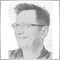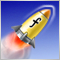Linear Regression Moving Average Algorithm312

//+------------------------------------------------------------------+\\
// Calculate LRMA
//+------------------------------------------------------------------+\\

double LRMA(const int pos,const int period,const double  &price[]) {

double Res=0;

double tmpS=0,tmpW=0,wsum=0;

for(int i=0;i<period;i++) {

tmpS+=price[pos+i];
tmpW+=price[pos+i]*(period-i);
wsum+=(period-i);
}

tmpS/=period;
tmpW/=wsum;
Res=3.0*tmpW-2.0*tmpS;

return(Res);
}

This is part of the code I downloaded from MT5 indicator code base.  Link is here.

I understand linear regression from this wiki page. If pos = 1 and period = 14, how the Res is calculated? Which formula does the LRMA() use?

for(int i=0;i<period;i++) {

tmpS+=price[pos+i];
tmpW+=price[pos+i]*(period-i);
wsum+=(period-i);
}

tmpS/=period;
tmpW/=wsum;
Res=3.0*tmpW-2.0*tmpS;

Can you explain the above code? My challenge is to understand the mathematical foundation of the above code.

Thanks!Color Linear Regression
• 2014.10.02
• Victor Nikolaev
• www.mql5.com
Color version of the Linear Regression indicator.2133

https://www.mql5.com/en/articles/2703 Methods of Indicators Acceleration by the Example of the Linear Regression
• 2011.08.04
• Andrew
• www.mql5.com
The article deals with the methods of indicators computational algorithms optimization. Everyone will find a method that suits his/her needs best. Three methods are described here.One of them is quite simple, the next one requires solid knowledge of Math and the last one requires some wit. Indicators or MetaTrader5 terminal design features are used to realize most of the described methods. The methods are quite universal and can be used not only for acceleration of the linear regression calculation, but also for many other indicators.312

good! I'll study it! Thank you!